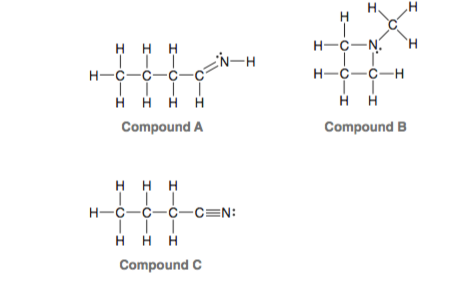# Problem: Consider the three compounds shown below and then answer the questions that follow: (h) Which compound do you predict will have the highest boiling point? Explain.

88% (84 ratings)
###### Problem Details

Consider the three compounds shown below and then answer the questions that follow:

(h) Which compound do you predict will have the highest boiling point? Explain.What scientific concept do you need to know in order to solve this problem?

Our tutors have indicated that to solve this problem you will need to apply the Intermolecular Forces concept. You can view video lessons to learn Intermolecular Forces. Or if you need more Intermolecular Forces practice, you can also practice Intermolecular Forces practice problems.

What is the difficulty of this problem?

Our tutors rated the difficulty ofConsider the three compounds shown below and then answer the...as medium difficulty.

How long does this problem take to solve?

Our expert Organic tutor, Jonathan took 1 minute and 21 seconds to solve this problem. You can follow their steps in the video explanation above.

What professor is this problem relevant for?

Based on our data, we think this problem is relevant for Professor Hyvl's class at HAWAII.

What textbook is this problem found in?

Our data indicates that this problem or a close variation was asked in Organic Chemistry - Klein 1st Edition. You can also practice Organic Chemistry - Klein 1st Edition practice problems.